# Geometry Solutions

Check the solutions for the Geometry Questions in detail along with shortcut techniques.

1) A Hexagon can be divided into equal parts as follows, leading to the formation of 12 equal triangles.

2) (c)

Deducing a Pattern: Taking two circles. The number of points of intersection is 2 at most. If u consider 3 circles, the number of points of intersection

= 6 ( the third circles will have 4 points of intersection with the other 2 circles and those two circles will have 2 points together)

Pattern = x(x-1)

Shortcut:- Assumption & Reverse Gear:

Taking two circles. The number of points of intersection is 2 at most. From this itself, option a, b and d can be eliminated giving the answer (c).

3) (c)

Angle PUT = 1000 ( VERTICALLY opposite) Since PR is the diagonal, <RPQ=45o < UTQ= 360-100-45-90 = 125

Thus <RTU= 180-125= 55.

4) (b) Assume a 45-45-90 triangle

Assume a 45-45-90 triangle

sin?a= P = 1/v2.

tana= Q = 1

1/P2+1/Q2 = 2-1 =1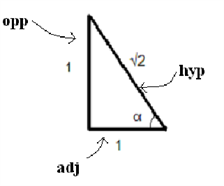5) (b)

Assume a 45-45-90 triangle. p=1 and q=1 Since only variables are used, the conditions should satisfy any right angled triangle. The side of the square becomes 0.5 or 1/2. The perimeter = 4× 1/2 = 2

Look at the answer options, where on the substitution of p=1 and q=1, we get 2. only option b gives 2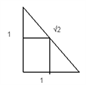6) (a)

Let the radius of the bigger circle= 1 Let the radius of the smaller circle = x A triangle can be formed with side= 2 Circumradius of the triangle = 2/3 x height = 2/3 x v3/2 x side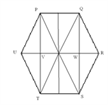(Area of hexagon)/((Area of PQRV) )=12/5

1+r= 2/3 x v3/2 x 2 r=2/v3-1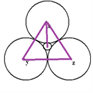Ratio of larger to the smaller ball = 1/(2/v3-1)=v3/(2-v3).

7) (c)

Draw the triangle with the width as its base and the third point on the opposite side. This triangle will have an area of lw/2. Even if we take the base as the length l still the area remains lw/2.

8) (c)

v140= 11.83.. This is a change of 2.17. i.e. 15%.

9) (a)

Two numbers are required who add up to 15 and whose squares add up to 153. Going from answer options, the only possibility is 12+3=15 and 144+9=153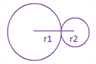So, Answer is 12/3= 4 = option (a)

10) (b).

In an equilateral triangle circum radius = 2/3 h, 10= 2/3*v3/2 s? Side = 103 units.

Area of Triangle = (v3)/4 x(10v3)2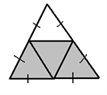By graphical division, we need ¾ th of the area of the triangle = 3/4 x (v3)/4 x (10v3)2 = (225v3)/4.

11) (b)Draw a square of side x and an arc of radius x.

Draw a square of side x and an arc of radius x.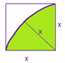All rectangles with diagonal less than or equal to x will lie within or on the quadrant of the circle. The shaded region is the favorable area. Hence, required probability = x2(1- p/4). (4-p)/4= 0.215= 21.5% chance.

12) (a) The quadrilateral formed by joining the mid-points of a rectangle is a rhombus.

The quadrilateral formed by joining the mid-points of a rectangle is a rhombus.

Area of rhombus = ½ ×area of rectangle

Area of inner rectangle = ½×(area of rhombus)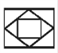Let the area of the biggest rectangle be 1 square unit. Therefore, Sum of area of all the rectangles obtained

= 1 +1/4+1/16+ … + a = 1/(1 -1/4)=4/3 square units

Sum of area of all the rhombuses

=1/2+1/8+1/32+….8=(1/2)/(1-1/4)=2/3

So, Required ratio=(4/3)/(2/3)=2:1

Hence (a).

13) (d)

If O is the center of the circle, and the radius is 4, AC will be the diameter of length 8 units. Hence, the answer is option (d).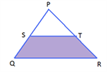14) No options.

Triangle PST & PQR are similar Thus PS/PQ=PT/PR => PR=8

Thus, PQR is a right angled triangle with sides-6,8,10 Area of trapezoid STQR= Area of triangle PQR- Area of triangle PST = 1/2×6×8 -1/2×3×4. The answer is 18 cm2.

15) (c)

If PQ=6, SY= 3 and QY= 3v3 (30-60-90 triangle)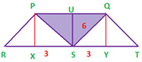Area of the trapezium = 1/2× (24)×3v3= 36v3

Area of the triangle = 1/2× 6 × 3v3= 9v3

Area of unshaded region = 36v3 – 9v3.

Ratio = 1:3.

16) (b)

Following the pattern

A1=p

A2=4p

A3=9p

A4=16p

Hence r=1,2,3,4

Thus C1= 2p C2= 4p C3= 6p C4= 8p. Ratio = 1.5. option (b).

17) (c)

Since the diagonals bisect at right angles, we can solve this question using the sum of squares concept discussed in Numbers.

The question boils down to this How many possibilities are there such that 92+a2=b2 where a,b are integers.There are only two possibilities

92+122=252 And 92+402=412

18) (d)

The resultant figure is made of three similar triangles. The height and radius will be in ratio 1/3:2/3:1.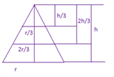Assume h=3

Volume of a cone= (1/3)pr2 h

1/3 p is common in each case, hence we do not need to consider that

Ratios = (27-8):( 8-1):1 = 19:7:1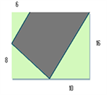19) (a)

Add the triangles (1/2× 6 × 8)+ (1/2× 8 × 6)+ (1/2× 10 × 16)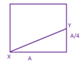20) (b)

Method 1:

Solving conventionally The string is wrapped equally around the cube 4 times. So the part ab = A/4. The hypotenuse of the triangle: v((A2 )+(A2/16) ). The length of the string is four times this hypotenuse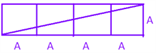4x(v17 A)/4= v17 A.

Hence Ans: b

Method 2:

Assume A=1, the thread will have to go around 4 times, hence its value will be greater than 4. It cannot be greater than 5 as well. Thus, answer is option (b)

Solving we get x=r/3 =AB/6 23) Since the only condition we need to maintain is that the diagonals need to intersect at right angles, assume the simplest quadrilateral which satisfies this condition- a square. Now the question can be solved by simple graphical division. Take the side of the square =p=q=2. Area = 4.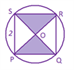The area of the shaded region is needed, which is half of that of the square= 2. Look in the answer options for 2 on substituting p=2 and q=2. The answer is the option (a).

24) (a)

The diameter of the circle = diagonal of the square = 2v2 Substituting in the answer options, one can get an answer as option (a).

25) (a)

This is a variable to variable question which can be easily solved by using numbers. As it is not mentioned anywhere that the diagram is drawn to scale, keeping in mind the preliminary conditions, we can redraw the figure as follows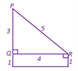area of this right angled triangle = ½ x base x height = ½ x 4 x 3= 6 The advantage of doing is that we can represent each variable in the form of numbers as follows p=1, q=4 and r=1.

Also, m=4 and n=0 substitute the values in the answer options and check where you are NOT getting 6. Options (b),(c),(d) and (e) are eliminated. Hence, Answer is the option (a).

Solution to Cube Problems:

26) (b)

The number of pieces obtained would be the maximum possible when the given numbers of cuts are made as equally as possible in the three parts. For n cuts, (n+1) pieces are obtained.

27) (a)

To get the minimum possible number of cuts when the given number of pieces is divided in such a way that factors are as equal as possible in three parts.

28) (a)

There is a pattern which can be applied to these questions. The number of cubes with 3 faces colours= 8 (All corner cubes).

Number of cubes have exactly two faces is painted = 12(n – 2)

Number of cubes have exactly one faces is painted = 6(n – 2)²

The number of cubes having no faces painted = (n – 2)³.  You can solve the above problem in no time at all. Here n=5 because 125=53 Here n=5

So, None of the faces painted= (n-2)³= 27.

To get more CAT practice questions on various topics, stay tuned with Byju’s. The candidates are also provided with all the other CAT exam related details along with the latest syllabus and pattern.